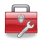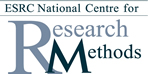# Using Statistical Regression Methods in Education Research# 4.9 Assumptions

Key Assumptions

You will find that the assumptions for logistic regression are very similar to the assumptions for linear regression. If you need a recap, rather than boring you by repeating ourselves like statistically obsessed parrots (the worst kind of parrot) we direct you to our multiple regression assumptions on Page 3.3. However, there are still three key assumptions which you should be aware of:

• Independent errors: Identical to linear regression, the assumption of independent errors states that errors should not be correlated for two observations. As we said before in the simple linear regression module, this assumption can often be violated in educational research where pupils are clustered together in a hierarchical structure. For example, pupils are clustered within classes and classes are clustered within schools. This means students within the same school often have a tendency to be more similar to each other than students drawn from different schools. Pupils learn in schools and characteristics of their schools, such as the school ethos, the quality of teachers and the ability of other pupils in the school, may affect their attainment. In large scale studies like the LSYPE such clustering can to some extent be taken care of by using design weights which indicate the probability with which an individual case was likely to be selected within the sample. Thus published analyses of LSYPE (see Resources Page) specify school as a cluster variable and apply published design weights using the SPSS complex samples module. More generally researchers can control for clustering through the use of multilevel regression models (also called hierarchical linear models, mixed models, random effects or variance component models) which explicitly recognise the hierarchical structure that may be present in your data. Sounds complicated, right? It definitely can be and these issues are more complicated than we need here where we are focusing on understanding the essentials of logistic regression. However if you feel you want to develop these skills we have an excellent sister website provided by another NCRM supported node called LEMMAwhich explicitly provides training on using multilevel modelling including for logistic regression. We also know of some good introductory texts on multilevel modelling and you can find all of this among our Resources.

• Multicollinearity: This is also identical to multiple regression. The assumption requires that explanatory variables should not be highly correlated with each other. Of course they are often correlated with each other to some degree. As an example, below is a correlation matrix (Figure 4.9.1) that shows the relationships between several LSYPE variables.

Figure 4.9.1: Correlation Matrix – searching for multicollinearity issues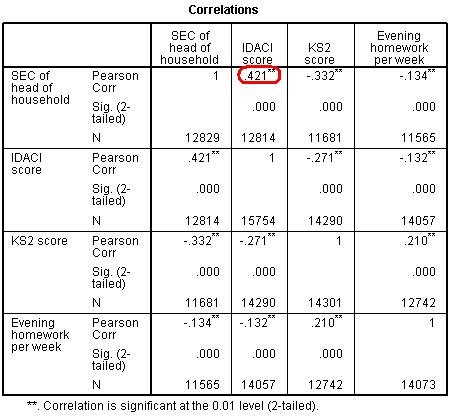As you might expect, IDACI (Income Deprivation Affecting Children Index) is significantly related to the SEC (socio-economic classification) of the head of the household but the relationship does not appear strong enough (Pearson’s r = .42) to be considered a problem. Usually values of r = .8 or more are cause for concern. As before the Variance Inflation Factor (VIF) and tolerance statistics can be used to help you verify that multicollinearity is not a problem (see Page 3.3).

 HomeModules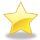Site Guide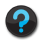Module 4 Contents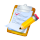Resources